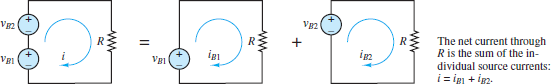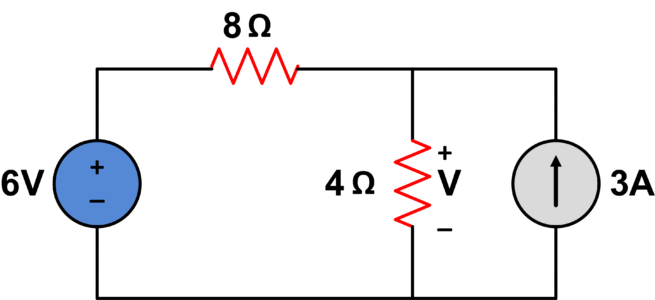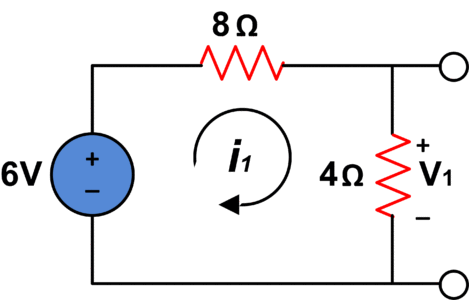# Superposition Theorem

Want create site? Find Free WordPress Themes and plugins.

The principle of superposition is a valid and frequently used, analytic tool for any linear circuit. It is also a powerful conceptual aid for understanding the behavior of circuits with multiple sources.

 For any linear circuit, the principle of superposition states that each independent source contributes to each voltage and current present in the circuit. Moreover, the contributions of one source are independent of those from the other sources. In this way, each voltage and each current in a circuit of N independent sources is the sum of N component voltages and N component currents, respectively.

As a problem-solving tool, the principle of superposition permits a problem to be decomposed into two or more simpler problems. The efficiency of this “divide and conquer” tactic depends upon the particular problem being solved. However, it may enable a simple closed-form solution of an otherwise complicated symbolic circuit problem, where node and mesh analyses may offer little help.

The method is to turn off (set to zero) all independent sources but one, and then solve for voltages and currents due to the lone remaining independent source. This procedure may be repeated successively for each source until the contributions due to all the sources have been computed. The components for a particular voltage or current can be summed to find its value in the original complete circuit.

A zero-voltage source is equivalent to a short circuit and a zero current source is equivalent to an open circuit. When using the principle of superposition, it is necessary, and helpful, to replace each zero source with its equivalent short- or open-circuit and thus simplify the circuit. These substitutions are summarized in Figure 1.

Superposition may be applied to circuits containing dependent sources; however, the dependent sources must not be set to zero. They are not independent sources and must not be treated as such. To do so, would lead to an incorrect result.Figure 1 Zeroing voltage and current sources

## Superposition Theorem Step by Step

1. Define the voltage V or current I to be solved in the circuit.

2. For each of the N sources, define a component voltage vk or current ik such that

$\begin{matrix}V={{v}_{1}}+{{v}_{2}}+…..+{{v}_{N}} & Or & I={{i}_{1}}+{{i}_{2}}+…..+{{i}_{N}} \\\end{matrix}$

3. Turn off all sources except source Sj and solve for the component voltage vk or current ik. Find components for all k where k = 1,2,…, N.

4. Find the complete solution for the voltage V or current I by summing all of the components as defined in step 2.

Details and Examples

An elementary application of the principle is to find the current in a single loop with two sources connected in series, as shown in Figure 2.Figure 2 The principle of superposition

The current in the far-left circuit of Figure 2 is easily found by a direct application of KVL and Ohm’s law.

$\begin{matrix}{{v}_{B1}}+{{v}_{B2}}-iR=0 & {} & {} \\Or & {} & \left( 1 \right) \\i=\frac{{{v}_{B1}}+{{v}_{B2}}}{R} & {} & {} \\\end{matrix}$

Figure 2 depicts the far-left circuit as being equivalent to the combined effects of two component circuits, each containing a single source. In each of these two circuits, one battery (which is a DC voltage source) has been set to zero and replaced with a short-circuit.

KVL and Ohm’s law can be applied directly to each of these component circuits.

$\begin{matrix}{{i}_{B1}}=\frac{{{v}_{B1}}}{R} & and & \begin{matrix}{{i}_{B2}}=\frac{{{v}_{B2}}}{R} & \left( 2 \right) \\\end{matrix} \\\end{matrix}$

According to the principle of superposition

$\begin{matrix}i={{i}_{B1}}+{{i}_{B2}}=\frac{{{v}_{B1}}}{R}+\frac{{{v}_{B2}}}{R}=\frac{{{v}_{B1}}+{{v}_{B2}}}{R} & {} & \left( 3 \right) \\\end{matrix}$

Voila! The complete solution is found, as expected. This simple example illustrates the essential method; however, more challenging examples are needed to reinforce it.

## Superposition Theorem Example

Find V in the circuit given below:After turning off current source, we haveUsing voltage divider;

${{V}_{1}}=\frac{4}{4+8}*6=2V$

Now, turning off the voltage source would give us the following result;Using current divider;

${{i}_{3}}=\frac{8}{4+8}*3=2A$

Whereas;

${{V}_{2}}=4*{{i}_{3}}=4*2=8V$

The algebraic sum of contribution from all sources would be;

$V={{V}_{1}}+{{V}_{2}}=2+8$

$V=10V$

Did you find apk for android? You can find new Free Android Games and apps.